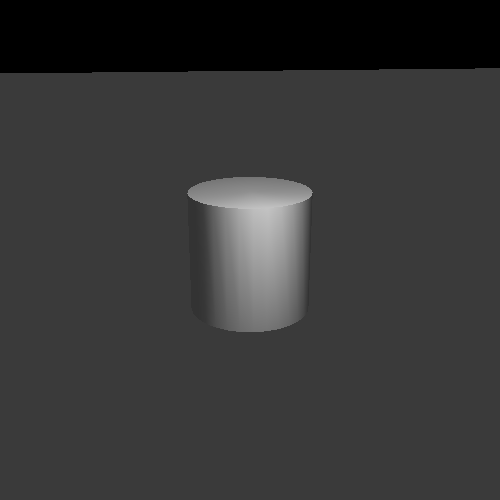## Chapter 10. Plane Lights

Directional lights are useful for representing light sources like the sun and so forth. But most light sources are more likely to be represented as point lights.

A point light source is a light source that has a position in the world and shines with equal intensity in all directions. Our simple diffuse lighting equation is a function of these properties:

• The surface normal at that point.

• The direction from the point on the surface to the light.

The direction to the light source from the point is a constant when dealing with directional light. It is a parameter for lighting, but it is a constant value for all points in the scene. The difference between directional lighting and point lights is only that this direction must be computed for each position in the scene.

Computing this is quite simple. At the point of interest, we take the difference between the point on the surface and the light's position. We normalize the result to produce a unit vector direction to the light. Then we use the light direction as we did before. The surface point, light position, and surface normal must all be in the same space for this equation to make sense.

## Vertex Point Lighting

Thus far, we have computed the lighting equation at each vertex and interpolated the results across the surface of the triangle. We will continue to do so for point lights. For the moment, at least.

We implement point lights per-vertex in the Vertex Point Lighting tutorial. This tutorial has a moving point light that circles around the cylinder.

Figure 10.1. Vertex Point LightingTo toggle an indicator of the light's position, press the Y key. The B key will toggle rotation of the light. The I and K keys move the light up and down respectively, while the J and L keys will decrease and increase the light's radius. Holding shift with these keys will move in smaller increments.

Most of the code is nothing we have not seen elsewhere. The main changes are at the top of the rendering function.

Example 10.1. Per-Vertex Point Light Rendering

```glutil::MatrixStack modelMatrix;
modelMatrix.SetMatrix(g_viewPole.CalcMatrix());

const glm::vec4 &worldLightPos = CalcLightPosition();

glm::vec4 lightPosCameraSpace = modelMatrix.Top() * worldLightPos;

glUseProgram(g_WhiteDiffuseColor.theProgram);
glUniform3fv(g_WhiteDiffuseColor.lightPosUnif, 1, glm::value_ptr(lightPosCameraSpace));
glUseProgram(g_VertexDiffuseColor.theProgram);
glUniform3fv(g_VertexDiffuseColor.lightPosUnif, 1, glm::value_ptr(lightPosCameraSpace));```

The light is computed initially in world space, then transformed into camera space. The camera-space light position is given to both of the shaders. Rendering proceeds normally from there.

Our vertex shader, `PosVertexLighting_PCN.vert` has had a few changes:

Example 10.2. Per-Vertex Point Light Vertex Shader

```#version 330

layout(location = 0) in vec3 position;
layout(location = 1) in vec4 diffuseColor;
layout(location = 2) in vec3 normal;

smooth out vec4 interpColor;

uniform vec3 lightPos;
uniform vec4 lightIntensity;
uniform vec4 ambientIntensity;

uniform mat4 modelToCameraMatrix;
uniform mat3 normalModelToCameraMatrix;

uniform Projection
{
mat4 cameraToClipMatrix;
};

void main()
{
vec4 cameraPosition = (modelToCameraMatrix * vec4(position, 1.0));
gl_Position = cameraToClipMatrix * cameraPosition;

vec3 normCamSpace = normalize(normalModelToCameraMatrix * normal);

vec3 dirToLight = normalize(lightPos - vec3(cameraPosition));

float cosAngIncidence = dot(normCamSpace, dirToLight);
cosAngIncidence = clamp(cosAngIncidence, 0, 1);

interpColor = (diffuseColor * lightIntensity * cosAngIncidence) +
(diffuseColor * ambientIntensity);
}```

The vertex shader takes a camera-space light position instead of a camera-space light direction. It also stores the camera-space vertex position in a temporary in the first line of `main`. This is used to compute the direction to the light. From there, the computation proceeds normally.

Note the order of operations in computing `dirToLight.` The `lightPos` is on the left and the `cameraPosition` is on the right. Geometrically, this is correct. If you have two points, and you want to find the direction from point A to point B, you compute B - A. The `normalize` call is just to convert it into a unit vector.